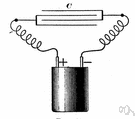# impedance

Also found in: Thesaurus, Medical, Legal, Financial, Acronyms, Encyclopedia, Wikipedia.

## im·ped·ance

(ĭm-pēd′ns)
n.
1. Symbol Z A measure of the total opposition to current flow in an alternating current circuit, made up of two components, ohmic resistance and reactance, and usually represented in complex notation as Z = R + iX, where R is the ohmic resistance and X is the reactance.
2. An analogous measure of resistance to an alternating effect, as the resistance to vibration of the medium in sound transmission.

## impedance

(ɪmˈpiːdəns)
n
1. (Electronics) a measure of the opposition to the flow of an alternating current equal to the square root of the sum of the squares of the resistance and the reactance, expressed in ohms. Symbol: Z
2. (Electronics) a component that offers impedance
3. (General Physics) Also called: acoustic impedance the ratio of the sound pressure in a medium to the rate of alternating flow of the medium through a specified surface due to the sound wave. Symbol: Za
4. (General Physics) Also called: mechanical impedance the ratio of the mechanical force, acting in the direction of motion, to the velocity of the resulting vibration. Symbol: Zm

## im•ped•ance

(ɪmˈpid ns)

n.
1. the total opposition to alternating current by an electric circuit, equal to the square root of the sum of the squares of the resistance and reactance of the circuit and usu. expressed in ohms.
2. the ratio of the force on a system undergoing harmonic motion to the velocity of the particles in the system.


## im·ped·ance

(ĭm-pēd′ns)
A measure of the opposition to the flow of an electric current through a circuit of alternating current. Impedance is measured in ohms.
ThesaurusAntonymsRelated WordsSynonymsLegend:
 Noun 1impedance - a material's opposition to the flow of electric current; measured in ohmselectrical phenomenon - a physical phenomenon involving electricityohmage - the ohmic resistance of a conductor
Translations
impedanssi
impedancija
impedancia

## impedance

[ɪmˈpiːdəns] N (Elec) →
References in periodicals archive ?
Applying a transmission line model based on the telegrapher's equations (as typically common in signal integrity considerations, except for when considering extremely high data rates, e.g., Serdes channels), one often-used general expression for the characteristic impedance of a lossy transmission line is:
Additionally, pre-stack inversion is useful for redeveloping brownfield 'as it gives more details about reservoir properties' through providing 'multiple impedance attributes and more resolution about lithology, porosity, and the fluid filling the pores,' as Shady Negm, CEO of Earthtec, told Egypt Oil and Gas.
The control performance and stability of the system are analysed by improving traditional voltage source impedance criterion to current source impedance ratio criterion.
Figure 2 shows the impedance response of the humidity sensors produced with varying PVDF nanofiber and ZnO mixtures.
Contributors from metrology and other scientific and engineering areas describe impedance spectroscopy and its advanced applications in battery research, bioimpedance, measurement systems, and sensors.
Conventional acoustic impedance (AI) inversion, which is the product of P-wave velocity and density, assumes that the P-wave strikes the subsurface interfaces at normal incidence (Lindseth, 1979).
"Impedance matching is basically trying to match the headphone to the music source or the player that you use.
There are two distinct MPP applications: (1), room acoustic applications, where high absorption coefficient is the target, and (2), duct and silencer applications, where surface impedance maximizing transmission loss (TL) is the target.
The next section introduces the theory of bioimpedance and electrical impedance spectroscopy.
In the patient-passive training, the movements are entirely actuated by the robot with position control, in which the robot is a mechanical admittance whereas the patient's arm is regarded as an impedance. Contrastively, in the patient-active training, movements are initiated and actuated by patients with partially assistance or resistance.
In , a folded step impedance resonator (SIR) was used for the NBPF, but the insertion loss was high.
To investigate the effects of elastic and plastic strains on eddy current response in metals by experimental tools, uniaxial tensile loading is commonly applied in the tensioned samples and the impedance variation of the eddy current sensor (ECS), which is placed near the sample surface, is recorded during the entire tension process .

Site: Follow: Share:
Open / Close